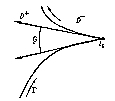# Sokhotskii formulas

Formulas first discovered by Yu.V. Sokhotskii , expressing the boundary values of a Cauchy-type integral. With more complete proofs but significantly later, the formulas were obtained independently by J. Plemelj .

Let $\Gamma$: $t= t( s)$, $0\leq s \leq l$, $t( 0)= t( l)$, be a closed smooth Jordan curve in the complex $z$- plane, let $\phi ( t)$ be the complex density in a Cauchy-type integral along $\Gamma$, on which $\phi ( t)$ satisfies a Hölder condition:

$$| \phi ( t _ {1} ) - \phi ( t _ {2} ) | \leq C | t _ {1} - t _ {2} | ^ \alpha ,\ 0< \alpha \leq 1;$$

let $D ^ {+}$ be the interior of $\Gamma$, $D ^ {-}$ its exterior, and let

$$\tag{1 } \Phi ( z) = \frac{1}{2 \pi i } \int\limits _ \Gamma \frac{\phi ( t) dt }{t-} z ,\ z \notin \Gamma ,$$

be a Cauchy-type integral. Then, for any point $t _ {0} \in \Gamma$ the limits

$$\Phi ^ {+} ( t _ {0} ) = \lim\limits _ {\begin{array}{c} z \rightarrow t _ {0} \\ z \in D ^ {+} \end{array} } \Phi ( z),$$

$$\Phi ^ {-} ( t _ {0} ) = \lim\limits _ {\begin{array}{c} z \rightarrow t _ {0} \\ z \in D ^ {-} \end{array} } \Phi ( z)$$

exist and are given by the Sokhotskii formulas

$$\tag{2 } \left . \begin{array}{c} \Phi ^ {+} ( t _ {0} ) = \frac{1}{2 \pi i } \int\limits _ \Gamma \frac{\phi ( t) dt }{t- t _ {0} } + \frac{1}{2} \phi ( t _ {0} ), \\ \Phi ^ {-} ( t _ {0} ) = \frac{1}{2 \pi i } \int\limits _ \Gamma \frac{\phi ( t) dt }{t- t _ {0} } - \frac{1}{2} \phi ( t _ {0} ), \\ \end{array} \right \}$$

or, equivalently,

$$\Phi ^ {+} ( t _ {0} ) + \Phi ^ {-} ( t _ {0} ) = \frac{1}{\pi i } \int\limits _ \Gamma \frac{\phi ( t) dt }{t- t _ {0} } ,$$

$$\Phi ^ {+} ( t _ {0} ) - \Phi ^ {-} ( t _ {0} ) = \phi ( t _ {0} ) .$$

The integrals along $\Gamma$ on the right-hand sides of these formulas are understood in the sense of the Cauchy principal value and are so-called singular integrals. By taking, under these conditions, $\Phi ^ {+} ( t)$( or $\Phi ^ {-} ( t)$) as values of the integral $\Phi ( z)$ on $\Gamma$, one thus obtains a function $\Phi ( z)$ that is continuous in the closed domain $\overline{ {D ^ {+} }}\; = D ^ {+} \cup \Gamma$( respectively, $\overline{ {D ^ {-} }}\; = D ^ {-} \cup \Gamma$). In the large, $\Phi ( z)$ is sometimes described as a piecewise-analytic function.

If $\alpha < 1$, then $\Phi ^ {+} ( t)$ and $\Phi ^ {-} ( t)$ are also Hölder continuous on $\Gamma$ with the same exponent $\alpha$, while if $\alpha = 1$, with any exponent $\alpha ^ \prime < 1$. For the corner points $t _ {0}$ of a piecewise-smooth curve $\Gamma$( see Fig.), the Sokhotskii formulas take the form

$$\tag{3 } \begin{array}{c} \Phi ^ {+} ( t _ {0} ) = \frac{1}{2 \pi i } \int\limits _ \Gamma \frac{\phi ( t) dt }{t- t _ {0} } + \left ( 1 - \frac \beta {2 \pi } \right ) \phi ( t _ {0} ), \\ \Phi ^ {-} ( t _ {0} ) = \frac{1}{2 \pi i } \int\limits _ \Gamma \frac{\phi ( t) dt }{t- t _ {0} } - \frac \beta {2 \pi } \phi ( t _ {0} ),\ 0\leq \beta \leq 2 \pi . \\ \end{array}$$

In the case of a non-closed piecewise-smooth curve $\Gamma$, the Sokhotskii formulas (2) and (3) remain valid for the interior points of the arc $\Gamma$.Figure: s086020a

The Sokhotskii formulas play a basic role in solving boundary value problems of function theory and in the theory of singular integral equations (see , ), and also in solving various applied problems in function theory (see ).

The question naturally arises as to the possibility of extending the conditions on the contour $\Gamma$ and the density $\phi ( t)$, in such a way that the Sokhotskii formulas (possibly with some restrictions) remain valid. The most significant results in this direction are due to V.V. Golubev and I.I. Privalov (see , ). For example, let $\Gamma$ be a rectifiable Jordan curve, and let the density $\phi ( t)$ be, as before, Hölder continuous on $\Gamma$. Then the Sokhotskii formulas (2) hold almost everywhere on $\Gamma$, where $\Phi ^ {+} ( t _ {0} )$ and $\Phi ^ {-} ( t _ {0} )$ are understood as non-tangential boundary values of the Cauchy-type integral from inside and outside $\Gamma$, respectively, but $\Phi ^ {+} ( z)$ and $\Phi ^ {-} ( z)$ are, in general, not continuous in the closed domains $\overline{ {D ^ {+} }}\;$ and $\overline{ {D ^ {-} }}\;$.

For spatial analogues of the Sokhotskii formulas see .

How to Cite This Entry:
Sokhotskii formulas. Encyclopedia of Mathematics. URL: http://encyclopediaofmath.org/index.php?title=Sokhotskii_formulas&oldid=49591
This article was adapted from an original article by E.D. Solomentsev (originator), which appeared in Encyclopedia of Mathematics - ISBN 1402006098. See original article